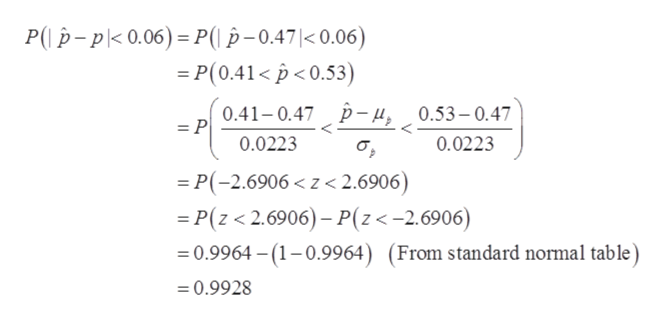# Suppose 47% of the population has a college degree.If a random sample of size 501 is selected, what is the probability that the proportion of persons with a college degree will differ from the population proportion by less than 6%? Round your answer to four decimal places.

Question
100 views

Suppose 47% of the population has a college degree.

If a random sample of size 501 is selected, what is the probability that the proportion of persons with a college degree will differ from the population proportion by less than 6%? Round your answer to four decimal places.

check_circle

Step 1

It is provided that

p = 0.47, q = 1- p = 1-0.47 = 0.53 and n = 501

Consider, the sampling distribution of sample proportion follows the normal distribution, that is,

Step 2

Thus,

Step 3

Now, the required probabilit...help_outlineImage TranscriptioncloseP(-pk0.06)- P( p-0.47|< 0.06) =P(0.41< <0.53) 0.41-0.47 p- 4, 0.53- 0.47 = P 0.0223 0.0223 P(-2.6906

### Want to see the full answer?

See Solution

#### Want to see this answer and more?

Solutions are written by subject experts who are available 24/7. Questions are typically answered within 1 hour.*

See Solution
*Response times may vary by subject and question.
Tagged in

### Other